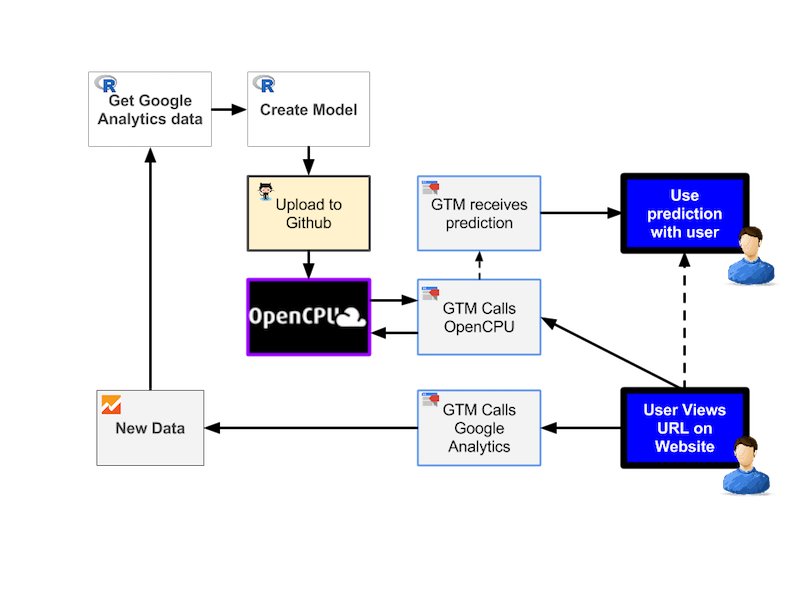6 March 2016

## Intro to R• Statistical programming language
• 2 million users. 40% increase last year
• Open Source = lots of cool libraries
• Libraries to collect, mung, visualise, machine learning, stats, etc. etc.
• Using R in a digital analytics workflow - my first Measurecamp presentation

## Intro to OpenCPU## Intro to Google Tag Manager• Free tag management system on Google infrastructure
• JavaScript container you can edit remotely
• DataLayer object to manage data in centralised manner
• Deploy analytics tracking, beacons or any JavaScript

## Data flow diagram## R - Creating the model

For our chosen model, need individual user session info e.g. need a userId

```library(googleAnalyticsR)
ga_auth()
gaId <- xxxx # Your View ID

## In this case, dimension3 contains userId in format:
## u={cid}&t={hit-timestamp}
start = "2016-02-01",
end = "2016-02-01",
metrics = c("pageviews"),
dimensions = c("dimension3", "pagePath"))```

For Adobe Analytics use the `RSiteCatalyst` library.

## Raw Data

dimension3 pagePath pageviews
u=100116318.1454322382&t=1454322382033 /example/809 1
u=100116318.1454322382&t=1454322412130 /example/1212 1
u=100116318.1454322382&t=1454322431492 /example/339 1
u=100116318.1454322382&t=1454322441120 /example/1494 1
u=100116318.1454322382&t=1454322450156 /example/339 1
u=100116318.1454322382&t=1454322461871 /example/1703 1

## Process GA data

Group per user the pages they visited in order of timestamp

```library(tidyr); library(dplyr)

## put dimension3: u={cid}&t={timestamp} in own columns "cid" and "timestamp"
processed <- raw %>% extract(col = dimension3,
into = c("cid","timestamp"),
regex = "u=(.+)&t=(.+)")
## javascript to R timestamp
processed\$timestamp <- as.POSIXct(as.numeric(processed\$timestamp) / 1000,
origin = "1970-01-01")

## find users with session length > 1 e.g. not a bounce visits
nonbounce <- processed %>% group_by(cid) %>%
summarise(session_length = n()) %>% filter(session_length > 1)
processed <- nonbounce %>% left_join(processed)```

## GA data - after processing

cid sessionLen timestamp pagePath pageviews
1005103157.1454327958 2 2016-02-01 11:59:18 /example/1 1
1005103157.1454327958 2 2016-02-01 12:02:42 /example/155 1
1010303050.1454327644 2 2016-02-01 11:54:03 /example/144 1
1010303050.1454327644 2 2016-02-01 12:00:03 /example/80 1
1011007665.1454333263 2 2016-02-01 13:27:43 /example/1359 1

## GA data - fit for model

Our model library `clickstream` needs a vector of sequential pageviews per userId. You may also need to aggregate the pages - unique for each website so not included here.

```## for each cid, make a string of pagePath in timestamp order
sequence <- processed %>% group_by(cid) %>%
summarise(sequence = paste(pagePath, collapse = ","))

sequence <- paste(sequence\$cid, sequence\$sequence, sep=",")```
```## example entries of page sequence per user for clickstream
example_sequence[]```
`##  "100116318.1454322382,/example/809,/example/1212,/example/339,/example/1494,/example/339,/example/1703,/example/1703,/example/1722,/example/1703"`

## Create Model

Create a Markov chain model of first order

```library(clickstream)

# fitting a simple Markov chain and predicting the next click
csf <- tempfile()
writeLines(sequence, csf)

## 1612 users - computing time: 285 seconds
model <- fitMarkovChain(cls, verbose=TRUE)

## save model for use on OpenCPU
save(model, file="./data/model.rda")```

## Using model for predictions

Almost instant predictions now we have built the model object.

```## see ?clickstream for details

## make predictions
> predict(model,
new("Pattern",
sequence = c("/example/96","/example/213","/example/107")))

## prediction output
Sequence: /example/251
Probability: 0.5657379
Absorbing Probabilities:
None
1    0```